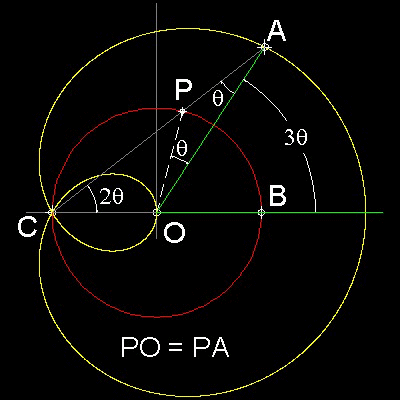Special Curves
Go to   Fun_Math Content Table   Trisecting an Angle   Special Curves

### Trisection using Special Curves

#### Limaçon- by Pascal

Limaçon was used by Blaise Pascal (1623 - 1662) for Angle Trisection.
His idea is shown in the figure shown below.##### Equation of the curve

In polar corrdinate: r = 2cos q + b

In rectangular coordinate: (x2 + y2 - 2x)2 = b2(x2 + y2)

##### Angle Trisection
Since Triangles COP and POA are isosceles,

Ð POA = Ð PAO and Ð OCP = Ð OPC = 2 x Ð PAO

Ð AOB = Ð PCO + Ð PAO = 3 x Ð PAO

Therefore Ð AOB is trisected.

******** limason_tri_desc.dwg ********

You can see the process in animation.

To create this drawing and animation: